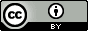## Saturday, February 28, 2015

### The 15N chemical shift of formamide. Is there an NMR expert in da house?

Update 2015.03.8: I have now talked to our local NMR expert and belives the 260.0 value more because the correction for bulk magnetic susceptibility are not always terribly accurate.

All I want (for now) is the value of the nitrogen chemical shift of formamide in water, but I found two very different values in Annual Reports on NMR Spectroscopy volume 11B: 263.7 and 267.8 ppm. The reference is nitromethane and for some reason it lists negative chemical shifts (i.e. $-\delta_x = \sigma_x - \sigma_{\mathrm{MeNO_2}}$).

The 263.7 value comes from this paper which reports a chemical shift of 260.0 ppm relative to saturated sodium nitrate which is then converted to the above value by adding the difference in chemical shift between sodium nitrate and nitromethane: 3.7 ppm.

The 267.7 value comes from this paper which reports a chemical shift of 261.6 ppm relative to 1 M nitric acid, which is then converted to the above value by adding the difference in chemical shift between 1 sodium nitrate and nitromethane plus a correction for the difference in bulk magnetic susceptibility ($\Delta\chi$): 4.4 + 1.8 = 6.2 ppm.  This extra correction ($\frac{4}{3}\pi(\chi_{\mathrm{MeNO_2}}-\chi_{\mathrm{H_2O}}$) is done because the experiment was performed with the external magnetic "field parallel to the sample tube".

The question is which value is the correct chemical shift for the nitrogen chemical shift of formamide in water?

Also, how hard would it be to measure it in the gas phase?